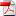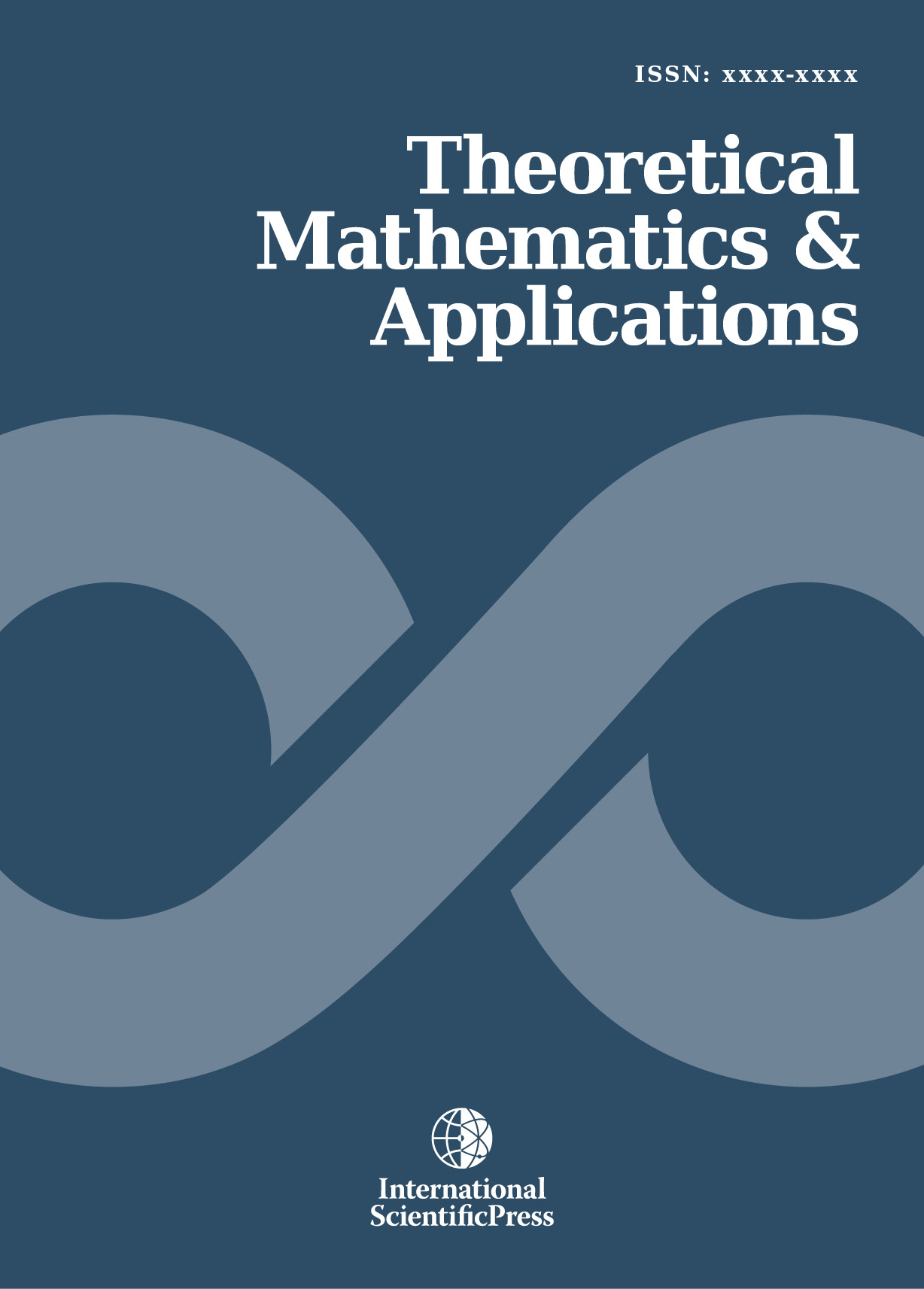# Theoretical Mathematics & Applications

#### A Mathematical Study of Electro-Magneto- Thermo-Voigt Viscoelastic Surface Wave Propagation under Gravity Involving Time Rate of Change of Strain

•[ Download ]
• Times downloaded: 1755
• Abstract

This research is a mathematical investigation of the propagation of surface wave in a Voigt viscoelastic medium. A mathematical model for wave propagation in electro-magneto-thermo heterogeneous viscoelastic isotropic half space under gravity involving time rate of change of strain of nth order is purposed. A solution to the partial differential equation of motion is assumed and is shown to satisfy the two necessary boundary conditions. The frequency equations for surface waves (Love, Rayleigh and Stoneley waves) are obtained with the help of Biot’s theory of incremental deformations. Heterogeneities in the medium are assumed to vary exponentially with depth. The problem investigated by Bullen [Cambridge University Press, pp. 85-99, 1965] has been reduced as particular case.ISSN: 1792-9687 (Print)
1792-9709 (Online)